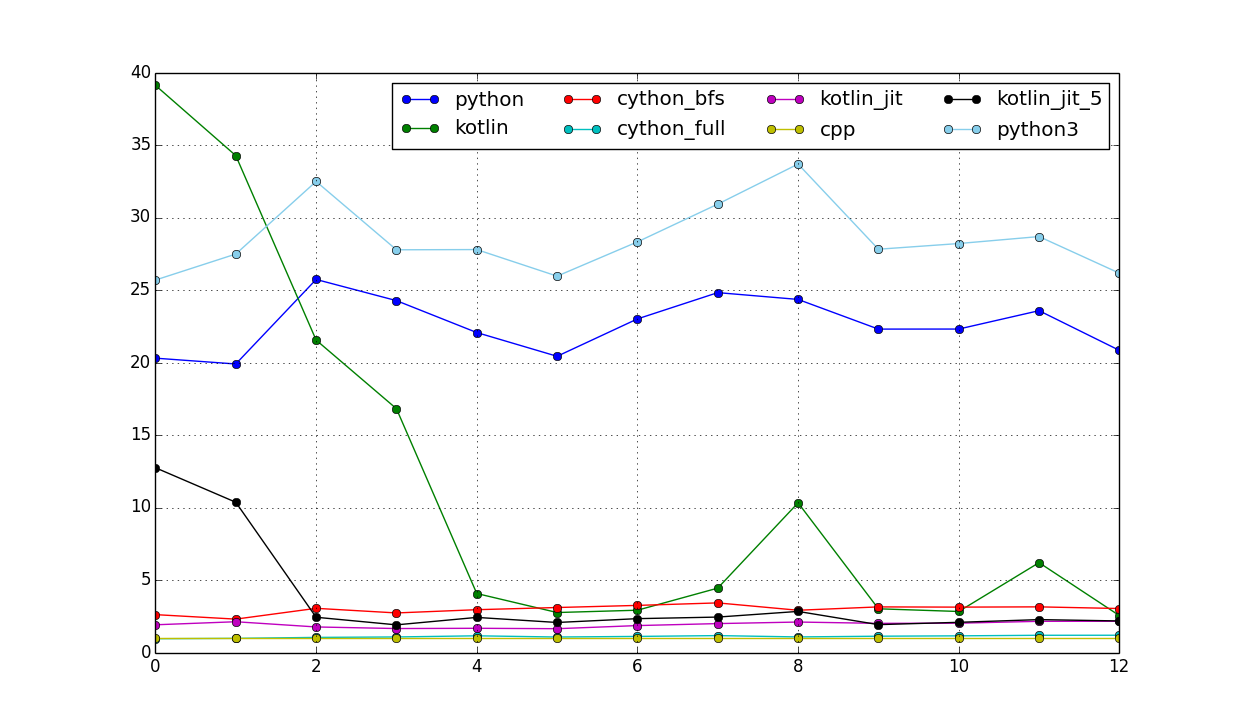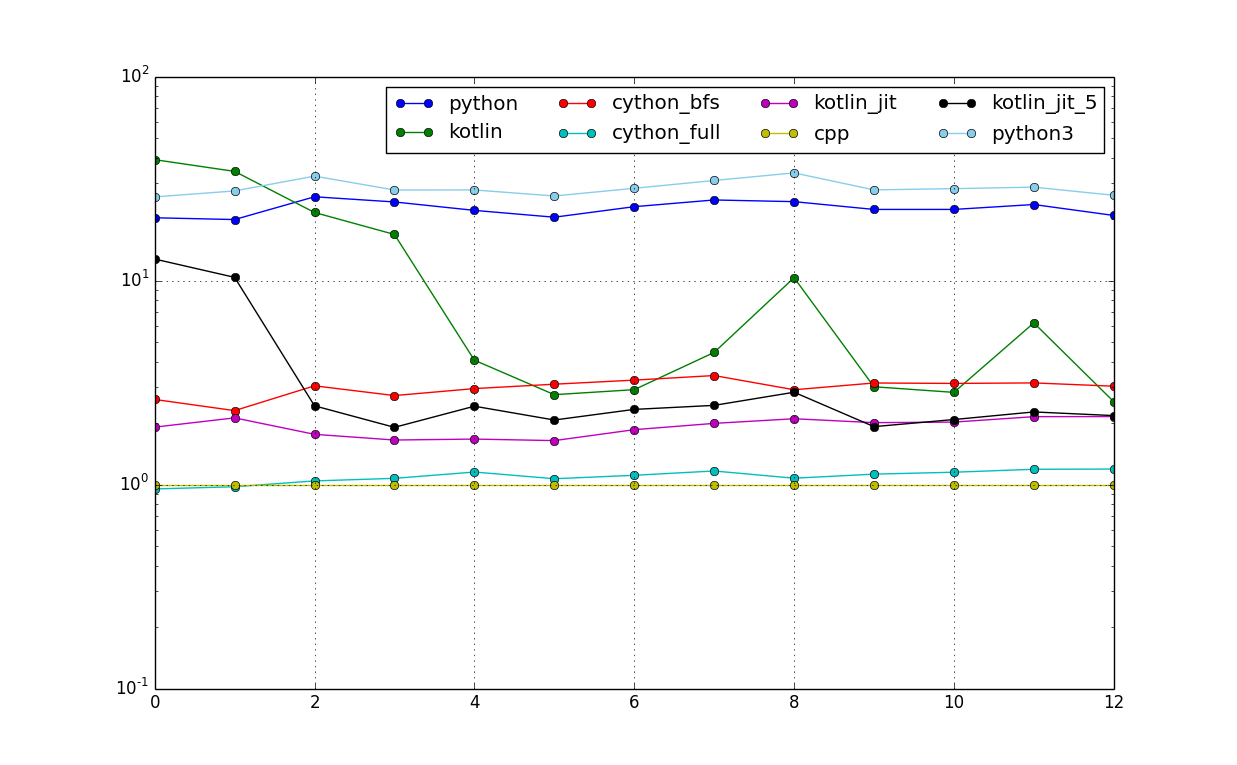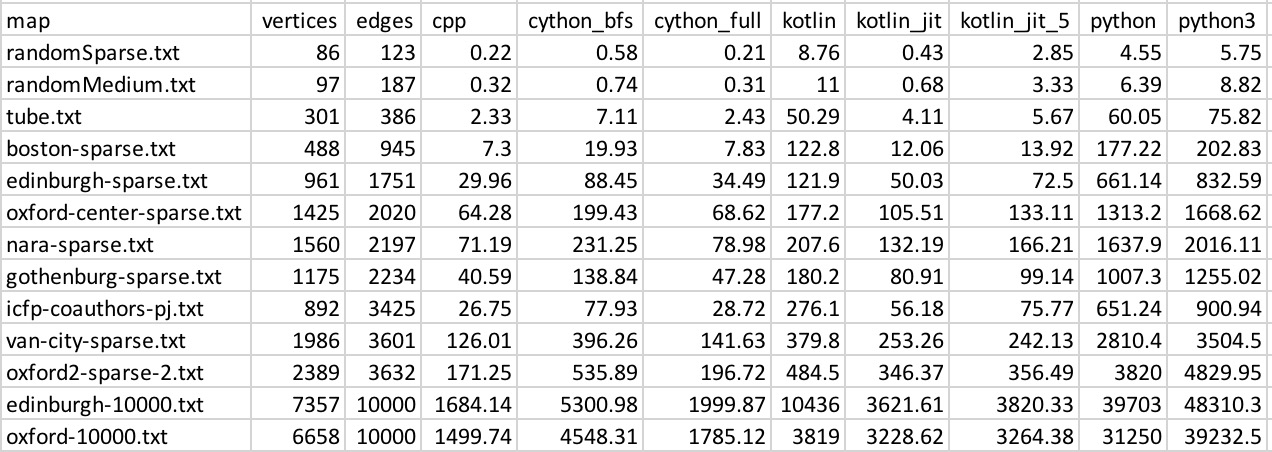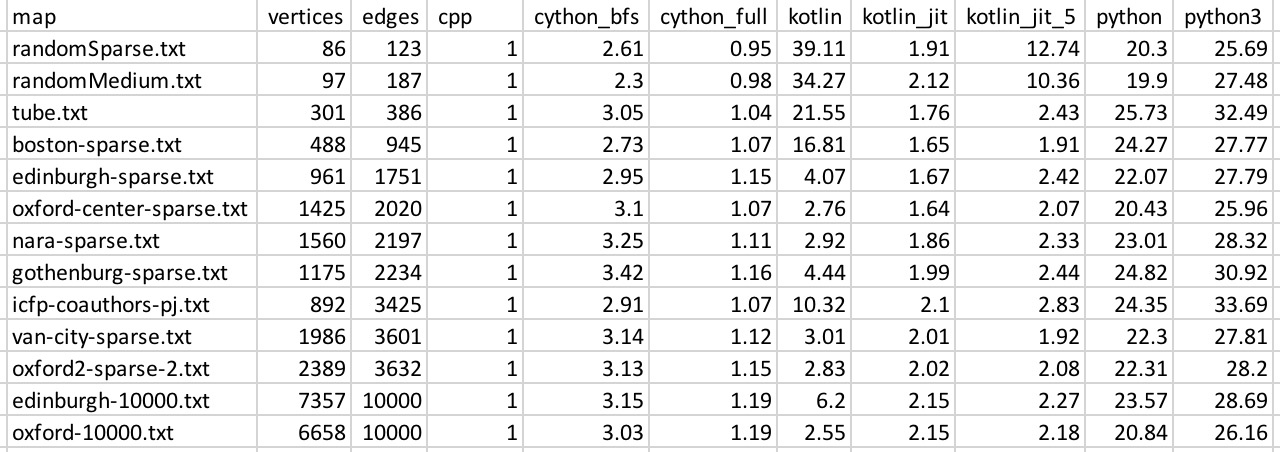In this note I want to compare performance of the following languages:

• `C++`
• `Kotlin`
• `Python`

One could argue that (obviously) `C++` is the fastest and `python` is the slowest. But I think it would be interesting to see some concrete numbers based on realistic benchmark, so here we go. Besides it turned out there are some interesting details that could slow down or speed up computations in certain cases.

# Short Summary

• Github link: https://github.com/pankdm/lang-perf
• `Python` is `~25x` slower than `C++`
• `Kotlin` is `~2x` slower than `C++`
• JIT in `Kotlin` can speed up the algorithm up to `30x`

# Methodology

For benchmark I used subproblem from the ICFPC-2017 contest where you need to do BFS on a graph. There graphs were ranging from 10 to 10000 edges. I filtered tiny ones and got a following list of 13 maps:

``````0: randomSparse.txt      	V=86	E=123
1: randomMedium.txt      	V=97	E=187
2: tube.txt              	V=301	E=386
3: boston-sparse.txt     	V=488	E=945
4: edinburgh-sparse.txt  	V=961	E=1751
5: oxford-center-sparse.txt	V=1425	E=2020
6: nara-sparse.txt       	V=1560	E=2197
7: gothenburg-sparse.txt 	V=1175	E=2234
8: icfp-coauthors-pj.txt 	V=892	E=3425
9: van-city-sparse.txt   	V=1986	E=3601
10: oxford2-sparse-2.txt  	V=2389	E=3632
11: edinburgh-10000.txt   	V=7357	E=10000
12: oxford-10000.txt      	V=6658	E=10000
``````

All languages implemented the same algorithm:

1. Read the graph from the file
2. From each node run BFS and save results
3. Compute graph “hash”:
``````for v in all_nodes:
tmp = 0
for u in all_nodes:
d = distance(v, u) # 0 if u is unreachable from v
tmp = tmp ^ (d * d) # xor
hash += tmp
return hash
``````

Step 1 wasn’t measured in total time to focus only on pure CPU work comparison (and avoid measuring IO and serialization/deserialization costs).

# Languages compared

1. `cpp`: C++ with -O3 optimization
2. `python`: python 2.7
3. `python3`: python 3.6
4. `cython_full`: cython with having both steps 2 and 3 implemented in C++
5. `cython_bfs`: cython with only bfs implemented in C++ (step 2)
6. `kotlin`: single run of Kotlin
7. `kotlin_jit_5`: run 5 times Kotlin program in a loop and measure the last run
8. `kotlin_jit`: run 100 times and measure last run

# Scripts

There are pre-requisites in being able to run scripts

``````# install cython:
pip install Cython

# install kotlin:
brew install kotlin
``````

Some of the languages require compilation before running, so the following command will prepare it:

``````\$ compile_all.sh
``````

Then the config in `bench.py` contains specification how to run each language

``````RUN_SCRIPTS = {
"cpp": "./cpp/run.sh",
"python": "./python/run.sh",
"python3": "./python/run_python3.sh",
"kotlin": "./kotlin/run.sh",
"kotlin_jit": "./kotlin/run_jit.sh",
"kotlin_jit_5": "./kotlin/run_jit_5.sh",
"cython_bfs": "./cython/run.sh",
"cython_full": "./cython/run_full.sh",
}
``````

This scripts will run benchmarks over available maps and append results into separate file `results.txt`:

``````{"map": "vancouver.txt", "edges": 3601, "graph_hash": "3987248", "time": "123.39", "nodes": 1986, "name": "cpp"}
...
``````

This file then could be visualized using the following command:

``````python visualize.py
``````

This will produce the graph of relative timings compared to c++:And also with logarithmic scale:# Results

## Absolute times (ms)## Relative times